Chapter 8. Electronic Structure

# Organization of Electrons in Atoms

Learning Objectives

1. Learn how electrons are organized in atoms.
2. Represent the organization of electrons by an electron configuration.

Now that you know that electrons have quantum numbers, how are they arranged in atoms? The key to understanding electronic arrangement is summarized in the : no two electrons in an atom can have the same set of four quantum numbers. This dramatically limits the number of electrons that can exist in a shell or a subshell.

Electrons are typically organized around an atom by starting at the lowest possible quantum numbers first, which are the shells–subshells with lower energies. Consider H, an atom with a single electron only. Under normal conditions, the single electron would go into the n = 1 shell, which has only a single s subshell with one orbital (because m can equal only 0). The convention is to label the shell–subshell combination with the number of the shell and the letter that represents the subshell. Thus, the electron goes in the 1s shell–subshell combination. It is usually not necessary to specify the m or ms quantum numbers, but for the H atom, the electron has m = 0 (the only possible value) and an ms of either +½ or −½.

The He atom has two electrons. The second electron can also go into the 1s shell–subshell combination but only if its spin quantum number is different from the first electron’s spin quantum number. Thus, the sets of quantum numbers for the two electrons are {1, 0, 0, +½} and {1, 0, 0, −½}. Notice that the overall set is different for the two electrons, as required by the Pauli exclusion principle.

The next atom is Li, with three electrons. However, now the Pauli exclusion principle implies that we cannot put that electron in the 1s shell–subshell because no matter how we try, this third electron would have the same set of four quantum numbers as one of the first two electrons. So this third electron must be assigned to a different shell–subshell combination. However, the n = 1 shell doesn’t have another subshell; it is restricted to having just ℓ = 0, or an s subshell. Therefore, this third electron has to be assigned to the n = 2 shell, which has an s (ℓ = 0) subshell and a p (ℓ = 1) subshell. Again, we usually start with the lowest quantum number, so this third electron is assigned to the 2s shell–subshell combination of quantum numbers.

The Pauli exclusion principle has the net effect of limiting the number of electrons that can be assigned a shell–subshell combination of quantum numbers. For example, in any s subshell, no matter what the shell number, there can be a maximum of only two electrons. Once the s subshell is filled up, any additional electrons must go to another subshell in the shell (if it exists) or to higher-numbered shell. A similar analysis shows that a p subshell can hold a maximum of six electrons. A d subshell can hold a maximum of 10 electrons, while an f subshell can have a maximum of 14 electrons. By limiting subshells to these maxima, we can distribute the available electrons to their shells and subshells.

Example 8.4

# Problem

How would the six electrons for C be assigned to the n and ℓ quantum numbers?

## Solution

The first two electrons go into the 1s shell–subshell combination. Two additional electrons can go into the 2s shell–subshell, but now this subshell is filled with the maximum number of electrons. The n = 2 shell also has a p subshell, so the remaining two electrons can go into the 2p subshell. The 2p subshell is not completely filled because it can hold a maximum of six electrons.

# Test Yourself

How would the 11 electrons for Na be assigned to the n and ℓ quantum numbers?

Two 1s electrons, two 2s electrons, six 2p electrons, and one 3s electron.

Now that we see how electrons are partitioned among the shells and subshells, we need a more concise way of communicating this partitioning. Chemists use an to represent the organization of electrons in shells and subshells in an atom. An electron configuration simply lists the shell and subshell labels, with a right superscript giving the number of electrons in that subshell. The shells and subshells are listed in the order of filling.

For example, an H atom has a single electron in the 1s subshell. Its electron configuration is: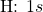He has two electrons in the 1s subshell. Its electron configuration is: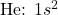The three electrons for Li are arranged in the 1s subshell (two electrons) and the 2s subshell (one electron). The electron configuration of Li is: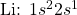Be has four electrons, two in the 1s subshell and two in the 2s subshell. Its electron configuration is:Now that the 2s subshell is filled, electrons in larger atoms must go into the 2p subshell, which can hold a maximum of six electrons. The next six elements progressively fill up the 2p subshell: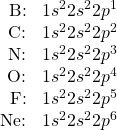Now that the 2p subshell is filled (all possible subshells in the n = 2 shell), the next electron for the next-larger atom must go into the n = 3 shell, s subshell.

Example 8.5

# Problem

What is the electron configuration for Na, which has 11 electrons?

## Solution

The first two electrons occupy the 1s subshell. The next two occupy the 2s subshell, while the next six electrons occupy the 2p subshell. This gives us 10 electrons so far, with 1 electron left. This last electron goes into the n = 3 shell, s subshell. Thus, the electron configuration of Na is 1s22s22p63s1.

# Test Yourself

What is the electron configuration for Mg, which has 12 electrons?

1s22s22p63s2

For larger atoms, the electron arrangement becomes more complicated. This is because after the 3p subshell is filled, filling the 4s subshell first actually leads to a lesser overall energy than filling the 3d subshell. Recall that while the principal quantum number largely dictates the energy of an electron, the angular momentum quantum number also has an impact on energy; by the time we get to the 3d and 4s subshells, we see overlap in the filling of the shells. Thus, after the 3p subshell is completely filled (which occurs for Ar), the next electron for K occupies the 4s subshell, not the 3d subshell: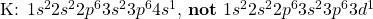For larger and larger atoms, the order of filling the shells and subshells seems to become even more complicated. There are some useful ways to remember the order, like that shown in Figure 8.08 “Electron Shell Filling Order.” If you follow the arrows in order, they pass through the subshells in the order that they are filled with electrons in larger atoms. Initially, the order is the same as the expected shell–subshell order, but for larger atoms, there is some shifting around of the principal quantum numbers. However, Figure 8.08 gives a valid ordering of filling subshells with electrons for most atoms.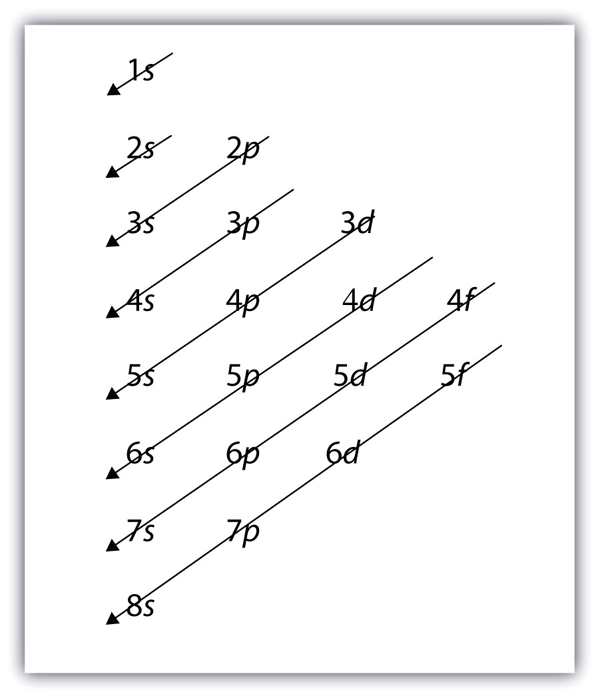Figure 8.08 “Electron Shell Filling Order.” Starting with the top arrow, follow each arrow. The subshells you reach along each arrow give the ordering of filling of subshells in larger atoms. The n = 5 and higher shells have more subshells, but only those subshells that are needed to accommodate the electrons of the known elements are given.

Example 8.6

# Problem

What is the predicted electron configuration for Sn, which has 50 electrons?

## Solution

We will follow the chart in Figure 8.08 “Electron Shell Filling Order” until we can accommodate 50 electrons in the subshells in the proper order: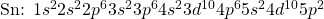Verify by adding the superscripts, which indicate the number of electrons:

2 + 2 + 6 + 2 + 6 + 2 + 10 + 6 + 2 + 10 + 2 = 50

Therefore, we have placed all 50 electrons in subshells in the proper order.

# Test Yourself

What is the electron configuration for Ba, which has 56 electrons?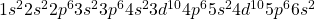As the previous example demonstrated, electron configurations can get fairly long. An  uses one of the elements from the last column of the periodic table, which contains what are called the noble gases, to represent the core of electrons up to that element. Then the remaining electrons are listed explicitly. For example, the abbreviated electron configuration for Li, which has three electrons, would be: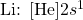Where [He] represents the two-electron core that is equivalent to He’s electron configuration. The square brackets represent the electron configuration of a noble gas. This is not much of an abbreviation. However, consider the abbreviated electron configuration for W, which has 74 electrons: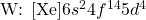This is a significant simplification over an explicit listing of all 74 electrons. So for larger elements, the abbreviated electron configuration can be a very useful shorthand.

Example 8.7

# Problem

What is the abbreviated electron configuration for P, which has 15 electrons?

## Solution

With 15 electrons, the electron configuration of P is: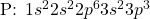The first immediate noble gas is Ne, which has an electron configuration of 1s22s22p6. Using the electron configuration of Ne to represent the first 10 electrons, the abbreviated electron configuration of P is: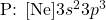# Test Yourself

What is the abbreviated electron configuration for Rb, which has 37 electrons?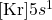There are some exceptions to the rigorous filling of subshells by electrons. In many cases, an electron goes from a higher-numbered shell to a lower-numbered but later-filled subshell to fill the later-filled subshell. One example is Ag. With 47 electrons, its electron configuration is predicted to be: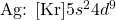However, experiments have shown that the electron configuration is actually: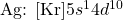This, then, qualifies as an exception to our expectations. At this point, you do not need to memorize the exceptions; but if you come across one, understand that it is an exception to the normal rules of filling subshells with electrons, which can happen.

# Electron Configuration Energy Diagrams

We have just seen that electrons fill orbitals in shells and subshells in a regular pattern, but why does it follow this pattern? There are three principles which should be followed to properly fill electron orbital energy diagrams:

1. The aufbau principle
2. The Pauli exclusion principle
3. Hund’s rule

The overall pattern of the electron shell filling order emerges from the (German for “building up”):  electrons fill the lowest energy orbitals first. Increasing the principle quantum number, n, increases orbital energy levels, as the electron density becomes more spread out away from the nucleus. In many-electron atoms (all atoms except hydrogen), the energy levels of subshells varies due to electron-electron repulsions. The trend that emerges is that energy levels increase with value of the angular momentum quantum number, l, for orbitals sharing the same principle quantum number, n. This is demonstrated in Figure 8.09, where each line represents an orbital, and each set of lines at the same energy represents a subshell of orbitals.

As previously discussed, the Pauli exclusion principle states that we can only fill each orbital with a maximum of two electrons of opposite spin. But how should we fill multiple orbitals of the same energy level within a subshell (e.g., the three orbitals in the 2p subshell)? Orbitals of the same energy level are known as degenerate orbitals, and we fill them using : place one electron into each degenerate orbital first, before pairing them in the same orbital. Let’s examine a few examples to demonstrate the use of the three principles.Boron is atomic number 5, and therefore has 5 electrons. First fill the lowest energy 1s orbital with two electrons of opposite spin, then the 2s orbital with 2 electrons of opposite spin and finally place the last electron into any of the three degenerate 2p orbitals (Figure 8.10).

Moving across the periodic table, we follow Hund’s rule and add an additional electron to each degenerate 2p orbital for each subsequent element (Figure 8.11). At oxygen we can finally pair up and fill one of the degenerate 2p orbitals.

Key Takeaways

• The Pauli exclusion principle limits the number of electrons in the subshells and shells.
• Electrons in larger atoms fill shells and subshells in a regular pattern that we can follow.
• Electron configurations are a shorthand method of indicating what subshells electrons occupy in atoms.
• Abbreviated electron configurations are a simpler way of representing electron configurations for larger atoms.
• Exceptions to the strict filling of subshells with electrons occur.
• Electron configurations are assigned from lowest to highest energy following the aufbau principle
• One electron is placed in each degenerate orbital before pairing electrons following Hund’s rule.
• Electron configuration energy diagrams follow three principles: the aufbau principle, the Pauli exclusion principle and Hund’s rule.

Exercises

# Questions

1. Give two possible sets of four quantum numbers for the electron in an H atom.
2. Give the possible sets of four quantum numbers for the electrons in a Li atom.
3. How many subshells are completely filled with electrons for Na? How many subshells are unfilled?
4. How many subshells are completely filled with electrons for Mg? How many subshells are unfilled?
5. What is the maximum number of electrons in the entire n = 2 shell?
6. What is the maximum number of electrons in the entire n = 4 shell?
7. Write the complete electron configuration for each atom.
1. Si, 14 electrons
2. Sc, 21 electrons
8. Write the complete electron configuration for each atom.
1. Br, 35 electrons
2. Be, 4 electrons
9. Write the complete electron configuration for each atom.
1. Cd, 48 electrons
2. Mg, 12 electrons
10. Write the complete electron configuration for each atom.
1. Cs, 55 electrons
2. Ar, 18 electrons
11. Write the abbreviated electron configuration for each atom in Exercise 7.
12. Write the abbreviated electron configuration for each atom in Exercise 8.
13. Write the abbreviated electron configuration for each atom in Exercise 9.
14. Write the abbreviated electron configuration for each atom in Exercise 10.
15. Draw electron configuration energy diagrams for potassium and bromine.

1. {1, 0, 0, +½} and {1, 0, 0, −½}
1. Three subshells (1s, 2s, 2p) are completely filled, and one shell (3s) is partially filled.
1. 8 electrons
1.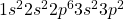2.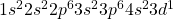1.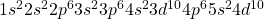2.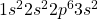1.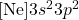2.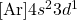1.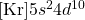2.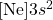1. Potassium:

Bromine:

# Long Descriptions

Figure 8.09 description: A blank diagram showing the different subshells of an atom and how many orbitals they contain. Remember that an orbital can hold two electrons. From lowest to highest energy, the subshells shown are:

• 1s (one orbital)
• 2s (one orbital)
• 2p (three orbitals)
• 3s (one orbital)
• 3p (three orbitals)

Figure 8.11 description: Electron configuration energy diagrams for carbon, nitrogen, and oxygen.

Carbon’s 1s and 2s subshells are full. In the 2p subshell, the first two orbitals have one electron each.

Nitrogen’s 1s and 2s subshells are full. In the 2p subshell, each of the three orbitals has one electron.

Oxygen’s 1s and 2s subshells are full. In the 2p subshell, the first orbital has two electrons, and the second and third orbitals have one electron each.